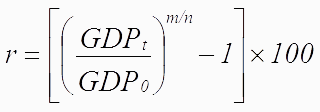The formula used by BEA to calculate the average annual growth is a variant of the compound interest formula:where

GDPt is the level of activity in the later period;

GDP0 is the level of activity in the earlier period;

m is the periodicity of the data (for example, 1 for annual data, 4 for quarterly data, or 12 for monthly data); and

n is the number of periods between the earlier period and the later period(that is t-0).

Published